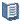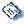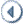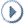Oracle® Fusion Applications Project Management Implementation Guide 11g Release 5 (11.1.5) Part Number E20384-05ContentsContactUsPreviousNext

# 24 Project Foundation Configuration: Define Labor Costing Business Unit Options

This chapter contains the following:

Overtime Calculation Components: How They Work Together

## Overtime Calculation Components: How They Work Together

If you charge overtime costs to a project, you can use Oracle Fusion Projects to record the cost premium that you pay for overtime. Your business can then recover overtime costs with higher bill rates or higher overhead rates.

You can set up Oracle Fusion Projects to calculate overtime hours and charge the hours to your overtime project using one of the following methods:

• Manually enter time card transactions to calculate overtime hours and charge them to a project.

• Implement the Overtime Calculation Extension to calculate and charge the hours to a project automatically.

Other components of overtime cost calculations are:

• Costing Method

• Overtime Expenditure Types

• Labor Costing Multipliers

• Labor Costing Rules

### Rates Costing Method

If you enter overtime hours manually, you can assign cost multipliers to overtime expenditure types. When you use the Rates costing method, and a transaction is charged to an expenditure type that has an assigned labor costing multiplier, the application applies the multiplier as labor costs are calculated. To calculate rates for overtime expenditure items, before you define labor costing rules, you must define an expenditure type with the Overtime expenditure type class.

When you charge overtime to the project on which overtime was worked, you can track all overtime costs on one expenditure item. Oracle Fusion Projects uses the following formula to calculate the overtime premium cost: ```(Overtime Hours * Hourly Cost Rate) + (Overtime Hours * Hourly Cost Rate * Labor Cost Multiplier)```.

### Extension Costing Method

Use the Overtime Calculation Extension to define your own rules to implement company-specific overtime calculation policies. The application calculates labor costs based on the logic that you configure in the extension. If you use this extension, labor costing rules establish the default overtime transaction attributes, such as business unit, project, and task. In addition, you are not required to maintain hourly cost rates in Oracle Fusion Projects.

Sample business rules in the Overtime Calculation Extension calculate and charge overtime costs to a project other than the project where the labor is charged. You can use this extension to create overtime transactions as separate expenditure items to track overtime costs. In addition, you can customize this extension to use multiple projects to track costs.

Note

To charge overtime to the project where the labor is charged, consider creating overtime expenditure items using the Related Transaction Extension.

If you use both the Overtime Calculation Extension and Related Transaction Extension, then you must define conditions in both extensions so that each transaction is processed by only one of the extensions.

Important

You must select the Enable Overtime Calculations business unit implementation option for the Import and Process Cost Transactions process to use the custom logic that you configure in the Overtime Calculation Extension to create overtime premium transactions.

### Overtime Expenditure Types

You must set up at least one overtime expenditure type that is classified by the Overtime expenditure type class.

Following are examples of overtime premium expenditure types and the corresponding expenditure type class:

Expenditure Type

Expenditure Type Class

Overtime

Overtime

### Labor Costing Multipliers

Labor costing multipliers are values by which labor cost rates are multiplied to calculate overtime premiums. You define a labor cost multiplier for each type of overtime your business uses, such as double time or time and a half. For example, if you pay a person double time for all overtime hours, then define a labor cost multiplier of 1.0. The person's labor cost rate is multiplied by 1.0 to calculate the person's overtime premium labor cost rate. If you pay a person time and a half for all overtime hours, then define a labor cost multiplier of 0.5 to calculate the person's overtime premium labor cost rate.

Oracle Fusion Projects uses the following formula to calculate the overtime premium cost rate: ```Labor Cost Rate * Labor Cost Multiplier = Overtime Premium Labor Cost Rate```. The application then multiplies the overtime premium labor cost rate by the number of overtime hours a person works to calculate the overtime premium for that person, as shown in this formula: ```Overtime Premium Labor Cost Rate * Overtime Hours = Overtime Premium Cost```.

A person's total labor cost is the overtime premium cost plus the total number of hours that the person worked multiplied by the person's labor cost rate, as shown in this formula: ```Overtime Premium Cost + (Total Hours * Labor Cost Rate) = Total Labor Cost```.

For example, assume that a person worked 10 hours of overtime at a rate of time and a half. The labor cost multiplier is 0.5, and the person's labor cost rate is \$40.00. Oracle Fusion Projects calculates the person's total labor cost as follows:

• \$40.00 * 0.5 = \$20.00 per hour Overtime Premium Labor Cost Rate

• \$20.00 * 10 hours = \$200.00 Overtime Premium Cost

• \$200.00 + (10 * \$40.00) = \$600.00 Total Labor Cost

### Labor Costing Rules

Labor costing rules associate overtime expenditure types with labor costing multipliers and determine how straight time and overtime costs are calculated. When a person charges time to a project, Oracle Fusion Projects processes the labor hours according to the person's labor costing rule.

You specify the default overtime project and overtime task in the labor costing rule to be used for the overtime expenditure items that are generated by the Overtime Calculation Extension.